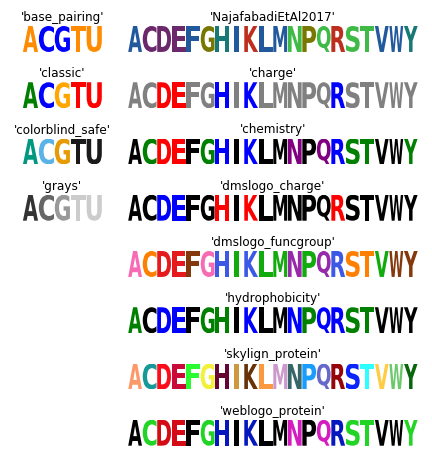# Examples¶

As described in Quick Start, the five logos shown in Figure 1 of Tareen and Kinney (2019)  can be generated using the function `logomaker.demo`. Here we describe each of these logos, as well as the snippets of code used to generate them. All snippets shown below are designed for use within a Jupyter Notebook, and assume that the following header cell has already been run.

```# standard imports
import numpy as np
import pandas as pd
import matplotlib.pyplot as plt

# displays logos inline within the notebook;
# remove if using a python interpreter instead
%matplotlib inline

# logomaker import
import logomaker
```

## Color schemes¶

The following code creates a figure that illustrates all of Logomaker’s built-in color schemes. To use one of these color schemes, set the `color_scheme` parameter to the indicated color scheme name when creating a `Logo` object.

```# get data frame of all color schemes
all_df = logomaker.list_color_schemes()

# set the two types of character sets
char_sets = ['ACGTU', 'ACDEFGHIKLMNPQRSTVWY']
colspans = [1, 3]
num_cols = sum(colspans)

# compute the number of rows
num_rows_per_set = []
for char_set in char_sets:
num_rows_per_set.append((all_df['characters'] == char_set).sum())
num_rows = max(num_rows_per_set)

# create figure
height_per_row = .8
width_per_col = 1.5
fig = plt.figure(figsize=[width_per_col * num_cols, height_per_row * num_rows])

# for each character set
for j, char_set in enumerate(char_sets):

# get color schemes for that character set only
df = all_df[all_df['characters'] == char_set].copy()
df.sort_values(by='color_scheme', inplace=True)
df.reset_index(inplace=True, drop=True)

# for each color scheme
for row_num, row in df.iterrows():
# set axes
col_num = sum(colspans[:j])
col_span = colspans[j]
ax = plt.subplot2grid((num_rows, num_cols), (row_num, col_num),
colspan=col_span)

# get color scheme
color_scheme = row['color_scheme']

# make matrix for character set
mat_df = logomaker.sequence_to_matrix(char_set)

# make and style logo
logomaker.Logo(mat_df,
ax=ax,
color_scheme=color_scheme,
show_spines=False)
ax.set_xticks([])
ax.set_yticks([])
ax.set_title(repr(color_scheme))

# style and save figure
fig.tight_layout()
```